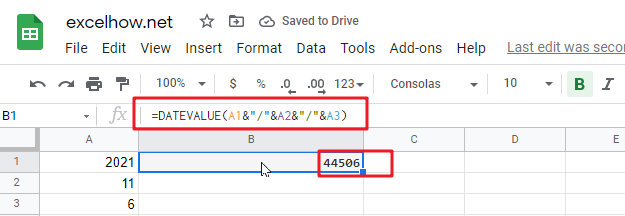This post will guide you how to use Google Sheets TODAY function with syntax and examples.

## Description

The Google Sheets TODAY function get the current date as a date value. And it will be updated each time when your worksheet is changed or opened. This function does not need to add any arguments.

You can use the TODAY function to get the current date displayed on your worksheet. And it also can be used for calculating intervals. For example, if you want to know that person’s age (he was born in 1984), you can use the following formula:

=YEAR(TODAY())-1984

This formula will use the TODAY function as an argument for the YEAR function to get the current year, and then subtracts 1984, getting his age.

The TODAY function is a build-in function in Google Sheets and it is categorized as a Date function.

## Syntax

The syntax of the TODAY function is as below:

=TODAY()

There are no any arguments for the TODAY function, but you still need to use empty parentheses ().

Note:

• TODAY function will be updated on every edit made to the spreadsheet and it will impact spreadsheet performance.
• TODAY function will create a date without the current time, and if you wish to get the current date and time, and you can use the NOW function.
• TODAY function will always get the current date and the last date in the spreadsheet was recalculated, rather than remaining at the date when it was first entered.

## Google Sheets TODAY Function Examples

The below examples will show you how to use google sheets TODAY Function to return the current system date.

1# using the TODAY function to get the current date, enter the following formula in Cell B1.

`=TODAY()`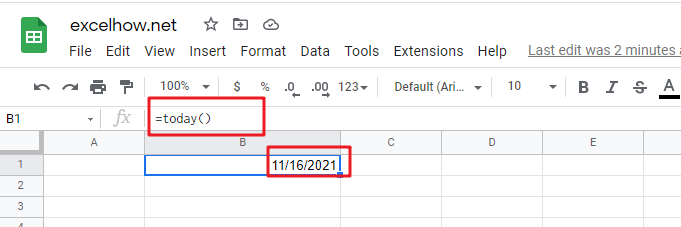2# Get the current date and then subtracts 10 days, using the following formula:

`=TODAY() -10`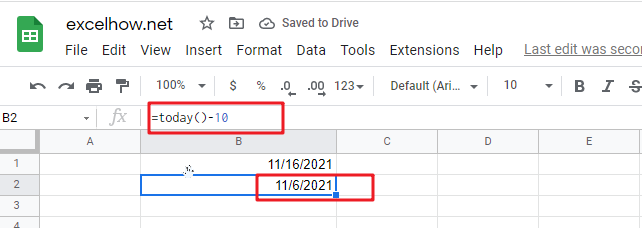3# Get the number of days between the current date and 03/14/2022, type the following formula:

`=DATEVALUE("03/14/2019")-TODAY()`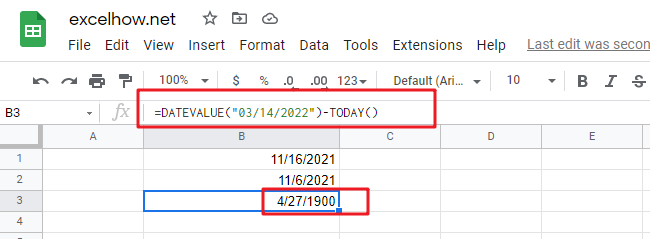4# Get the current day of the month, using the following formula:

`=DAY(TODAY())`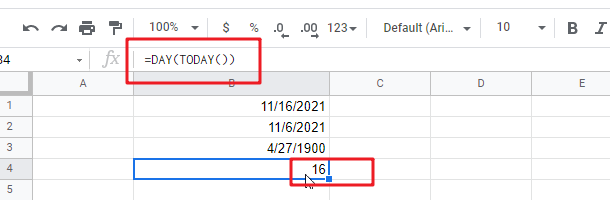5# Get the current month of the year, type the following formula in Cell B1:

`=MONTH(TODAY())`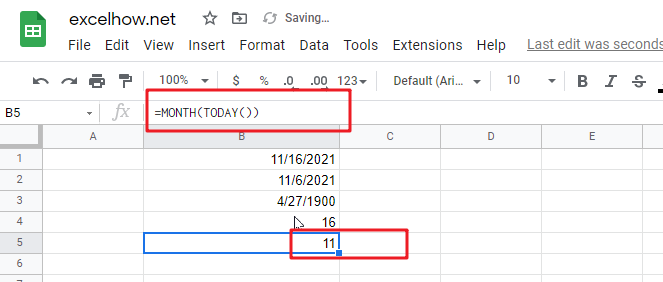This post will guide you how to use Google Sheets NETWORKDAYS.INTL function with syntax and examples.

## Description

The Google Sheets NETWORKDAYS.INTL function returns the number of net working days between tow given days excluding specified weekend days and holidays.

The NETWORKDAYS.INTL function can be used to calculate the number of working days between dates in google sheets. And NETWORKDAYS.INTL will exclude weekends optionally and provides a way to specify which days of the week should be considered as weekends.

The purpose of this function is to get the number of working days between two dates and its returned values is a number that representing days.

This function is more complex that the NETWORKDAYS function because it allows you to control which days of the week are considered weekends.

The NETWORKDAYS.INTL function is a build-in function in Google Sheets and it is categorized as a Date function.

## Syntax

The syntax of the NETWORKDAYS.INTL function is as below:

=NETWORKDAYS.INTL (start_date, end_date, [weekend], [holidays])

Where the NETWORKDAYS.INTL function argument is:

• Start_date -This is a required argument. The starting of date from which to calculate the number of working days.
• End_date – this is a required argument. The ending of date from which to calculate the number of working days.
• Weekend – this is an optional argument. A number that representing which days of the week are considered as weekends.
• Holidays – this is an optional argument. A list of non-working days.

Note:

• `Weekend` argument can be specified using seven 0’s and 1’s, where the first number represents Monday and the last number represents Sunday. A 0’s means that the day is a working day, and a 1’s means that the day is a weekend. For example, “0000011” means that Saturday and Sunday are weekends.
• `Weekend` argument also can be specified as a single number, you can refer to the below table displaying the weekend numbers and their corresponding weekend days.
 Weekend Number Weekend Days 1 or omitted Saturday and Sunday 2 Sunday and Monday 3 Monday and Tuesday 4 Tuesday and Wednesday 5 Wednesday and Thursday 6 Thursday and Friday 7 Friday and Saturday 11 Sunday only 12 Monday only 13 Tuesday only 14 Wednesday only 15 Thursday only 16 Friday only 17 Saturday only

• NETWORKDAYS.INTL function only calculate all workdays, ignoring any time values.
• NETWORKDAYS.INTL function will exclude all Saturday and Sunday automatically.
• NETWORKDAYS.INTL function must be included both the starting date and ending date when calculating workdays.
• Holidays argument must be a date serial number values, or a date value returned by N function, or returned by DATE, DATEVALUE functions. It should be standard date values or date serial numbers.
• If `start_date` is greater than `end_date`, NETWORKDAYS.INTL function will return a negative value.
• If `start_date` or `end_date` are out of range, NETWORKDAYS.INTL function will return the #NUM! error.

## Google Sheets NETWORKDAYS.INTL Function Examples

The below examples will show you how to calculate working days between two given dates, taking into account the specified weekends and holidays in google sheets.

#1 Calculate working days between two dates in range B1:B2 by excluding default weekends with weekend string value, see the below formula:

`=NETWORKDAYS.INTL(B1,B2,"1000000",C1:C4) //returns 295, exclude holidays, and Monday is weekend`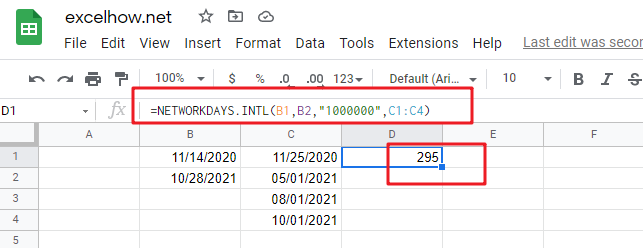The weekend string value “1000000” means that Monday is the weekend, and all other days are considered as working days.

This post will guide you how to use Google Sheets NETWORKDAYS function with syntax and examples.

## Description

The Google Sheets NETWORKDAYS function returns the number of net working days between tow given days.

The NETWORKDAYS function can be used to calculate the number of working days between dates in google sheets. And NETWORKDAYS will exclude weekends automatically. The purpose of this function is to get the number of working days between two dates and its returned values is a number that representing days.

The NETWORKDAYS function is a build-in function in Google Sheets and it is categorized as a Date function.

## Syntax

The syntax of the NETWORKDAYS function is as below:

=NETWORKDAYS (start_date, end_date, [holidays])

Where the NETWORKDAYS function argument is:

• Start_date -This is a required argument. The starting of date from which to calculate the number of working days.
• End_date – this is a required argument. The ending of date from which to calculate the number of working days.
• Holidays – this is an optional argument. A list of non-working days.

Note:

• NETWORKDAYS function only calculate all workdays, ignoring any time values.
• NETWORKDAYS function will exclude all Saturday and Sunday automatically.
• NETWORKDAYS function must be include both the starting date and ending date when calculating workdays.
• `Holidays` argument must be a date serial number values, or a date value returned by N function, or returned by DATE, DATEVALUE functions. It should be standard date values or date serial numbers.
• If you wish to use other days of week as the weekend, you can use the NETWORKDAYS.INTL function.

## Google Sheets NETWORKDAYS Function Examples

If you want to calculate the number of workdays between two dates in google sheets, you can use a formula based on the NETWORKDAYS function. And it will exclude all weekend days between the start dates and end dates. You need to specify two dates contain start date and end date so that you can get the number of workdays.

For example, you have two dates in range B1:B2, and you want to get the workdays between these two dates, you just need to use the following formula:

`=NETWORKDAYS(B1,B2)`

Type this formula in a blank Cell, and press Enter key in your keyboard.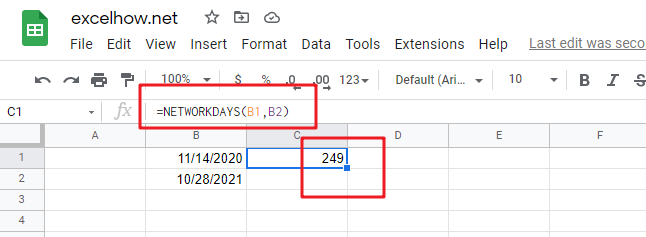The above formula contains the NETWORKDAYS function but does not take into account holidays, if you wish to take into account holidays( a range in C1:C4), just using the following formula:

`=NETWORKDAYS(B1,B2,C1:C4)`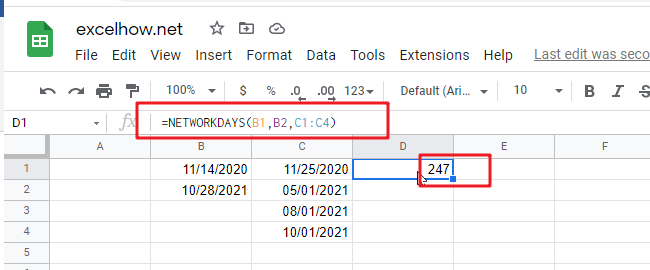This post will guide you how to use Google Sheets MONTH function with syntax and examples.

## Description

The Google Sheets MONTH function returns the month of a date represented by a serial number. And the month is an integer number from 1 to 12. And you can use the MONTH function to get a month component from a given date value. And you can feed the month number to another formula.

The MONTH function can be used to extract the month from a given date as a number between 1 to 12 in google sheets. The purpose of this function is to get MONTH as a number from a date and its returned values is a number between 1 and 12.

The MONTH function is a build-in function in Google Sheets and it is categorized as a Date function.

## Syntax

The syntax of the MONTH function is as below:

=MONTH (date)

Where the MONTH function argument is:

• Date -This is a required argument. A valid date value that contains the month component you want to extract. Dates should be entered by using the DATE function, or as results of other formulas or functions. For example, use DATE (2021,3,15) for the 15th day of March, 2021.

Note:

• You need to make sure that the Date argument is a cell reference that containing a date, or a function which returns a date value or a date serial number.
• If `Date` argument is not recognized, MONTH function will return #VALUE! Error message.
• If `Date` argument is supplied as a date number that is out of range, MONTH function will return #NUM! error message.
• You can use the MONTH function to extract a month component from a given date, or using this function to feed a month number into another function to create a more complex formula like the DATE function.
• Date argument can be supplied to the MONTH function as a date text string (e.g. “2021/11/13” or “Nov-11-2021”).  Or creating a date value with separate year, month, and day by using the DATE function.

## Google Sheets MONTH Function Examples

The below examples will show you how to use google sheets MONTH Function to return an integer number that representing the month component of a given date.

1# get month of the date in Cell A1, enter the following formula in Cell B1.

`=MONTH(A1)`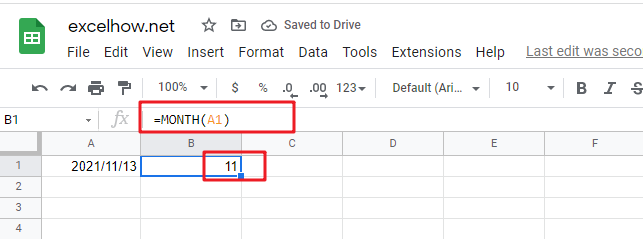2# convert Month name to number, type the following formula in Cell B1.

You should use the DATEVALUE function to convert a date stored as text to a serial number. Then feed the result into the MONTH function to extract a month number from date.

`=MONTH(DATEVALUE(A1 & "1"))`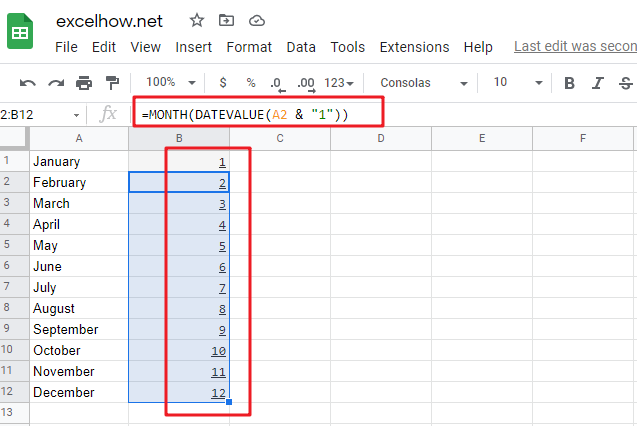This post will guide you how to use Google Sheets DATEVALUE function with syntax and examples.

## Description

The Google Sheets DATEVALUE returns the serial number of date and it can be used to convert a date represented as text format into a serial number that recognizes as a date format.

The DATEVALUE function can be used to convert a given date string in a known format to a date value. The purpose of this function is to convert a date in text format to a valid date and its returned values is a date value.

For example, you can convert a date “2021/11/6” that is text format to a serial number, you can use the formula: =DATEVALUE (“2021/11/6”). Then it returns a serial number “44506” you can use the google sheets dates to manipulate with formulas or pivot table to filter, sort. It is very useful than the text dates.

The DATEVALUE function is a build-in function in Google Sheets and it is categorized as a Date function.

## Syntax

The syntax of the DATEVALUE function is as below:

=DATEVALUE(date_text)

Where the DATEVALUE function arguments is:

• Date_text -This is a required argument. A valid date that the format is text. Or the text that represents a date in an excel date format.

Note:

• The date_text argument must represent a date between January 1, 1900 and December 31, 9999. The DATEVALUE function returns the #VALUE! Error value if the value of the date_text argument falls outside of this range.
• If the year portion of the date_text argument is omitted, the DATEVALUE function uses the current year from your computer’s built-in clock. Time information in the date_text argument is ignored.
• January 1 1900 is serial number 1 by default.
• Date_text argument must be a string, if a number or cell reference to a cell containing a number is provided, the DATEVALUE function will return the #VALUE! Error.
• If you want to provide an explicit string input to DATEVALUE function rather than a cell reference, you must surround quotation marks for inputting string.

## Google Sheets DATEVALUE Function Examples

The below examples will show you how to use google sheets DATEVALUE Function to return a serial number of a given date.

1# Get a serial number of a date (11/06/2021) the format as text, enter the following formula in Cell B1.

`=DATEVALUE("11/06/2021")`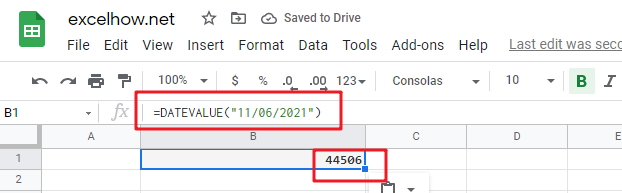2# Get a serial number of a date (6-Nov-2021) the format as text, enter the following formula in Cell B2.

`=DATEVALUE("6-Nov-2021")`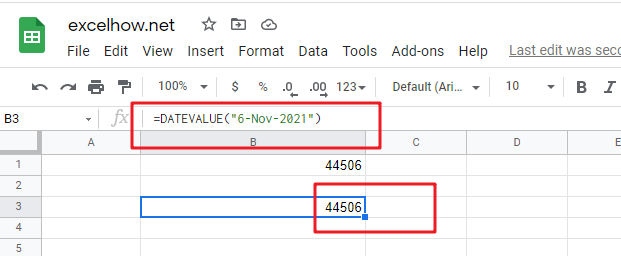3# Get a serial number of a date (2021/03/14) the format as text, enter the following formula in Cell B3.

`=DATEVALUE("2021/11/6")`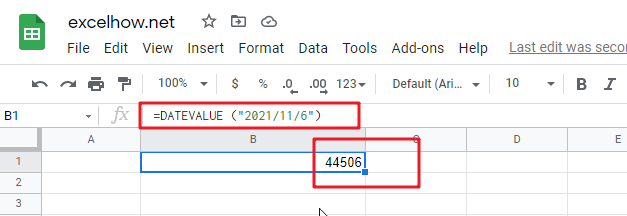4# get a serial number from a date that the year portion is omitted (11/6) assuming the current year is 2021, using the following formula:

`=DATEVALUE("11/6")`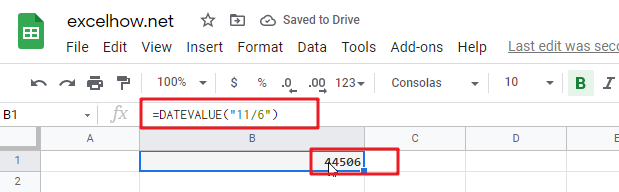5# get a serial number from a date that created by combining the values in Cells A1, A2, and A3

`=DATEVALUE(A1&"/"&A2&"/"&A3)`